﻿ 一种附加尺度因子的电离层层析新方法文章快速检索 高级检索
 大地测量与地球动力学2018, Vol. 38Issue (12): 1285-1290  DOI: 10.14075/j.jgg.2018.12.014### 引用本文LIU Tianyi, MING Feng, HONG Jun. A New Algorithm for Ionosphere Tomography by Using Scaling Factor[J]. Journal of Geodesy and Geodynamics, 2018, 38(12): 1285-1290.### Foundation support

National Natural Science Foundation of China, No. 61571417.

### About the first author

LIU Tianyi, postgraduate, majors in InSAR calibration, E-mail: liutianyi15@mails.ucas.ac.cn.

### 文章历史

1. 中国科学院电子学研究所，北京市北四环西路19号, 100190;
2. 微波成像技术重点实验室，北京市北四环西路19号, 100190;
3. 中国科学院大学，北京市玉泉路19号甲，100049

1 代数重构方法

 ${\rm{STEC}} = \int_l {{N_e}(s){\rm{d}}s}$ (1)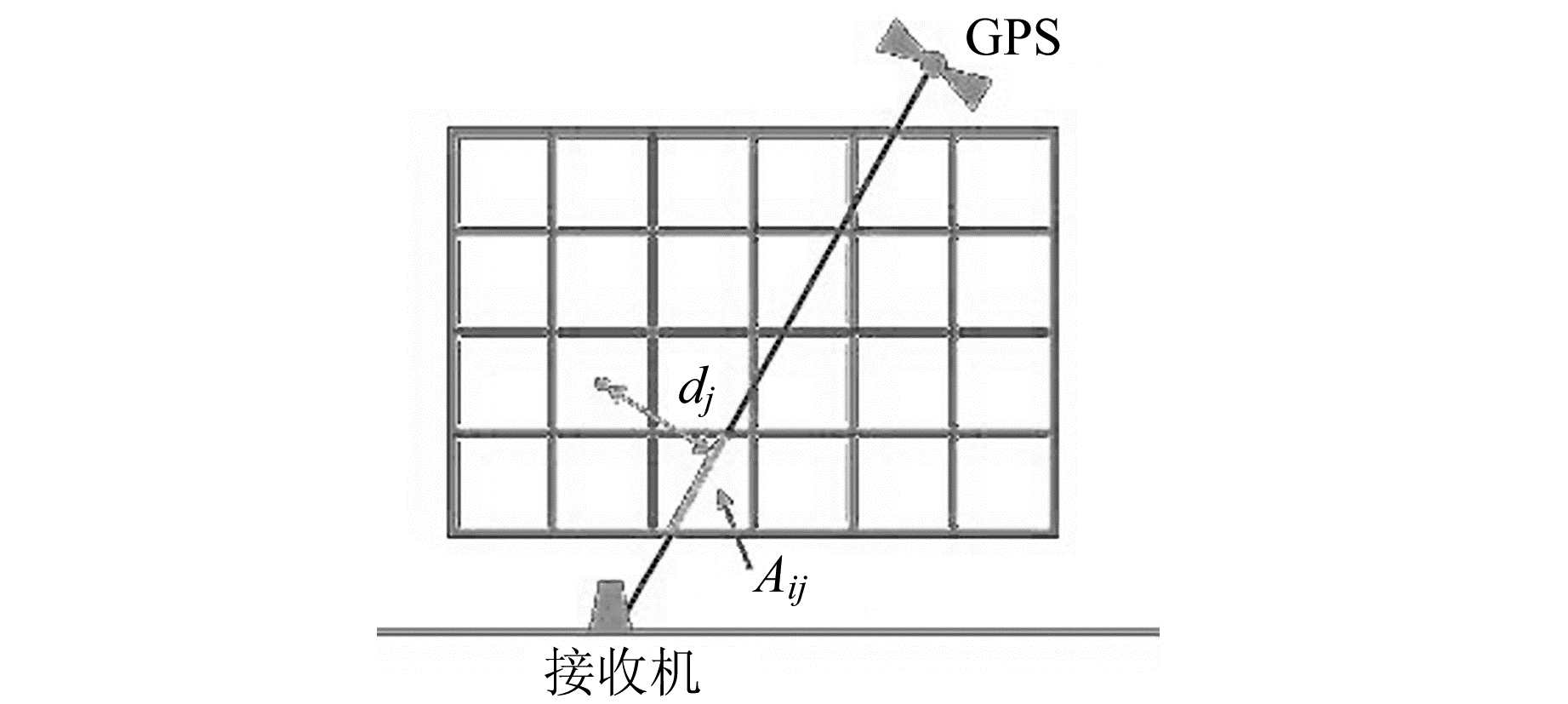图 1 GPS测量层析成像原理示意图 Fig. 1 Principle of GPS-based tomography in pixel model

 ${\rm{STE}}{{\rm{C}}_i} = \sum\limits_{j = 1}^n {{A_{ij}}{x_j} + {e_i}, i = 1, 2, ..., m}$ (2)

 ${\mathit{\boldsymbol{y}}_{m \times 1}} = {\mathit{\boldsymbol{A}}_{m \times n}}{\mathit{\boldsymbol{x}}_{n \times 1}} + {\mathit{\boldsymbol{e}}_{m \times 1}}$ (3)

Pryse等提出代数重构(ART)算法：

 $x_j^{k + 1} = x_j^k + {\lambda _k}\frac{{{y_i} - \sum\limits_{j = 1}^n {{A_{ij}}x_j^k} }}{{{{\left\| {{A_i}} \right\|}^2}}}{A_i}$ (4)

 $x_j^{k + 1} = x_j^k{\left( {{y_i}/\sum\limits_{j = 1}^n {{A_{ij}}x_j^k} } \right)^{\frac{{{\lambda _k}{A_{ij}}}}{{{\rm{max}}{A_{ij}}}}}}$ (5)
2 改进重构方法

 $x_j^{k + 1} = \left\{ \begin{array}{l} {s_{ij}}^kx_j^k, {A_{ij}} = 0\\ x_j^k + {\lambda _k}\frac{{{y_i} - \sum\limits_{j = 1}^n {{A_{ij}}x_j^k} }}{{{{\left\| {{A_i}} \right\|}^2}}}{A_i}, {A_{ij}} \ne 0 \end{array} \right.$ (6)

 $s_{i0}^k = \frac{{{y_i}}}{{\sum\limits_{j = 1}^n {{A_{ij}}x_j^k} }}$ (7)

 $s_{ij}^k = f({d_{ij}})$ (8)

 $f({d_{ij}}) = {\rm{exp}}(a{d_{ij}} + b)$ (9)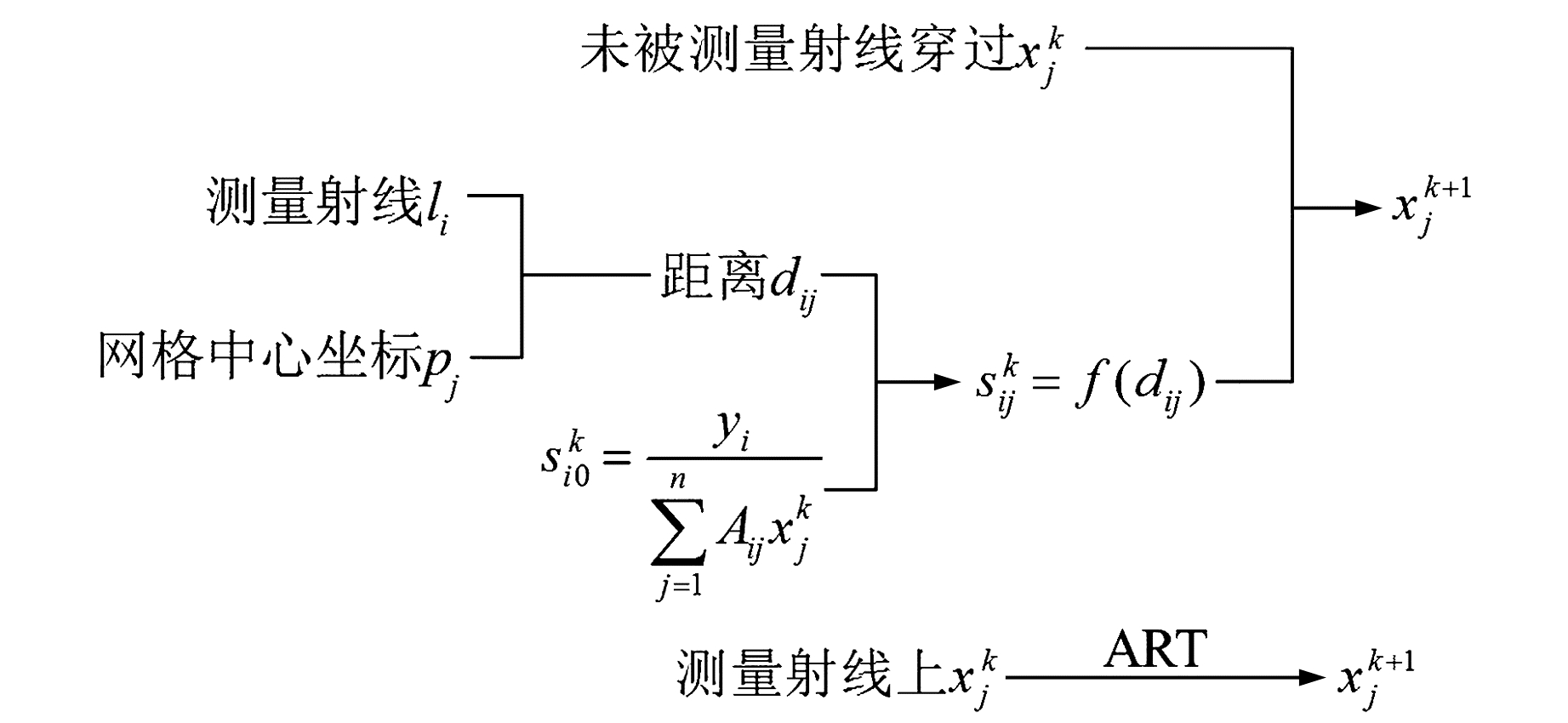图 2 Scaling-ART算法流程 Fig. 2 Flowchart of Scaling-ART algorithm
3 实验验证

3.1 数值模拟实验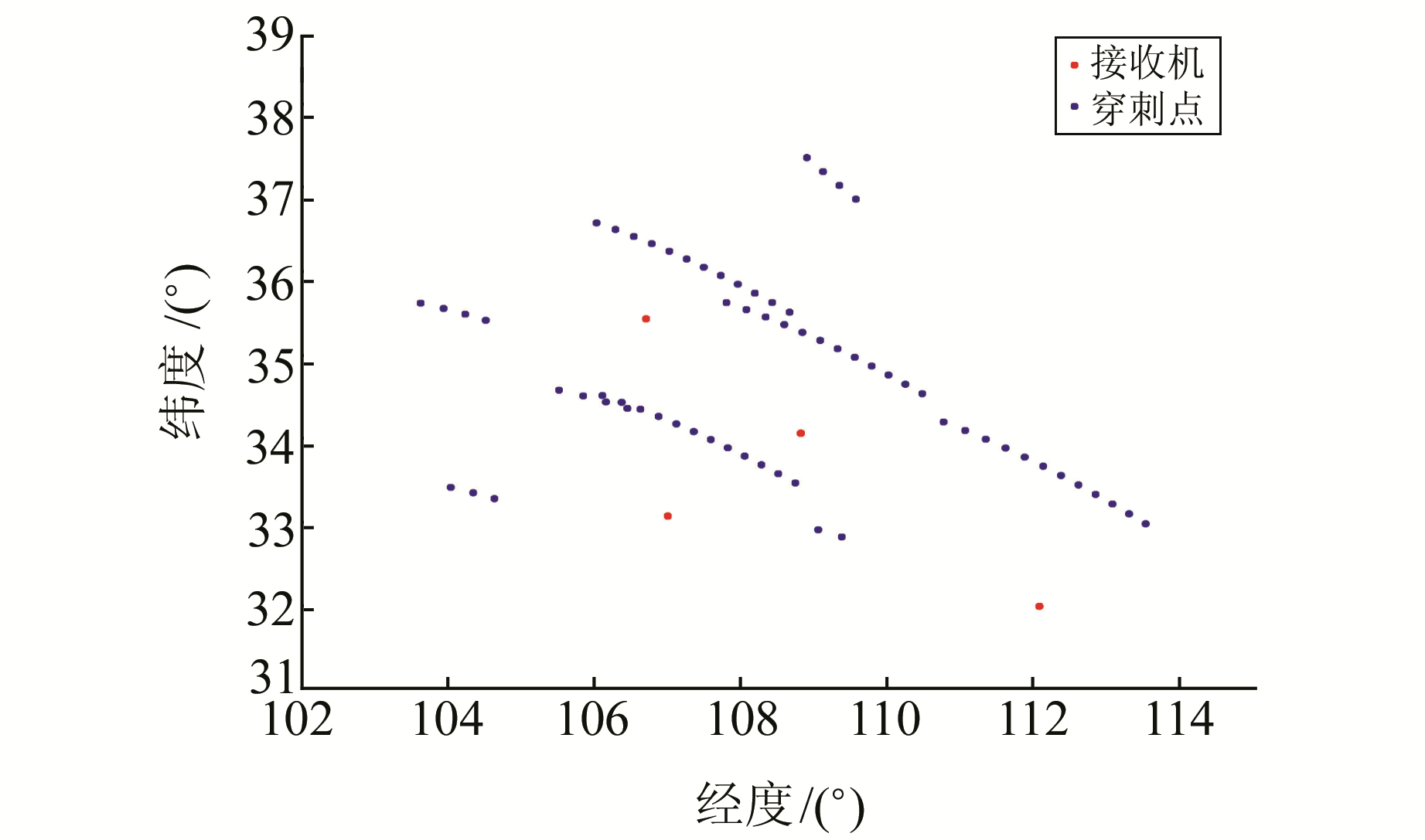图 3 接收机以及穿刺点坐标 Fig. 3 Position of receiver and ionospheric pierce points

STEC通过“真值”数据和截距矩阵得到。考虑到STEC测量时存在误差，生成STEC时加入一定量的随机噪声，噪声在[-10%, 10%]内均匀分布。图 4是IRI模型在100°E子午面的电子密度分布情况，电子密度峰值在300~350 km高度，随纬度增加电子密度呈现减小的趋势。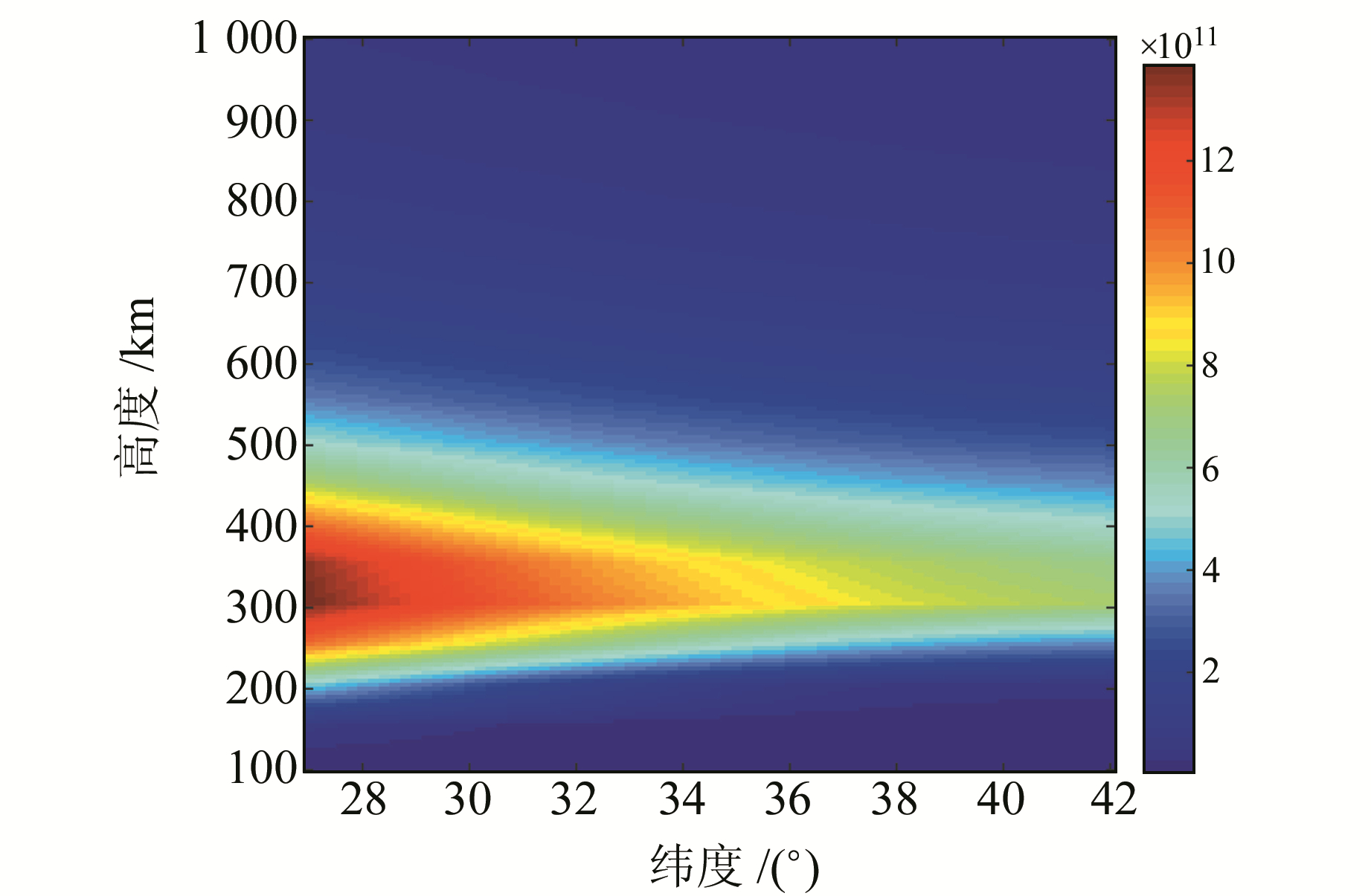图 4 IRI模型电子密度剖面分布 Fig. 4 IED image of IRI model图 5 尺度因子空间分布 Fig. 5 Image of spatial distribution of scaling factor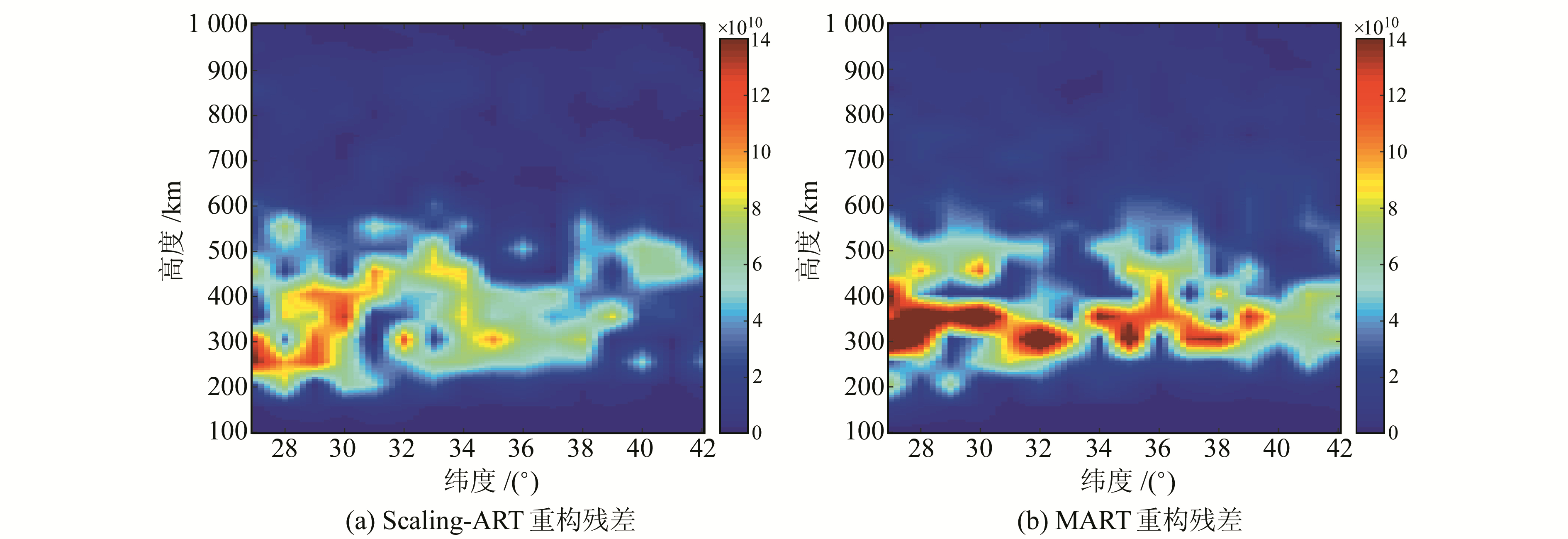图 6 2种算法同一经度反演残差对比 Fig. 6 Comparison of IED residual by MART and Scaling-ART vertical across along longitude图 7 2种算法不同纬度反演残差比较 Fig. 7 Comparison of IED residual by MART and Scaling-ART vertical across along different latitude

 ${\rm{RMSE}} = \sqrt {\frac{{\sum\limits_{j = 1}^n {({x_{r, j}} - {x_{e, j}})} }}{n}}$ (10)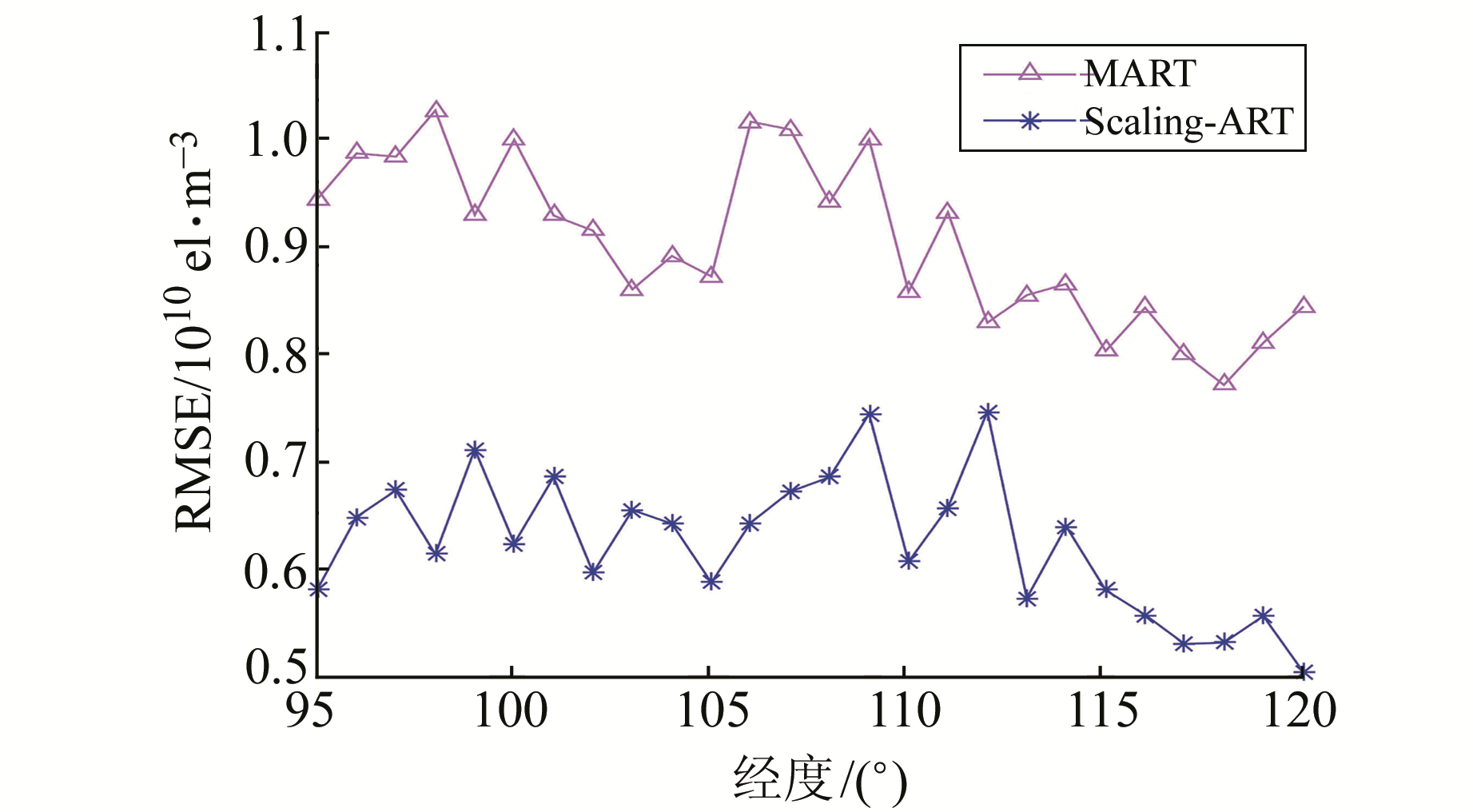图 8 各个经度反演RMSE Fig. 8 RMSE of IED at various longitude

3.2 实测数据验证

 ${\rm{dif}} = {\rm{er}}{{\rm{r}}_{{\rm{MART}}}} - {\rm{er}}{{\rm{r}}_{{\rm{Scaling - ART}}}}$ (11)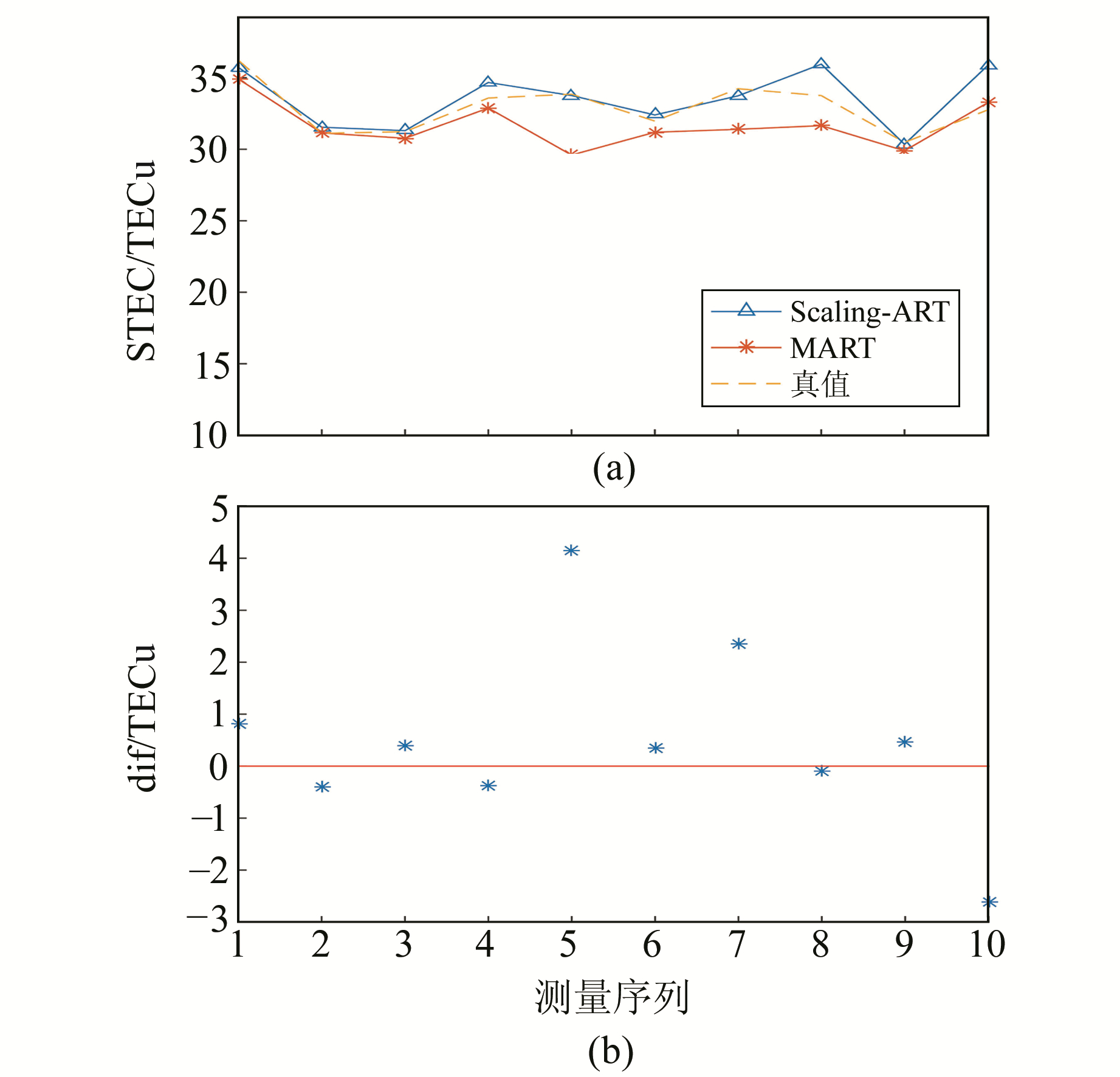图 9 MART算法与Scaling-ART算法STEC绝对误差比较 Fig. 9 Comparison of absolute STEC error of two algorithms
4 结语

  李亮, 洪峻, 明峰. 电离层对中高轨SAR影响机理研究[J]. 雷达学报, 2017, 6(6): 619-629 (Li Liang, Hong Jun, Ming Feng. Mechanism Study of Ionospheric Effects on Medium-Earth-Orbit SAR[J]. Journal of Radars, 2017, 6(6): 619-629) (0)  聂兆生, 祝芙英, 付宁波, 等. Kalman滤波在地震电离层TEC异常探测中的应用[J]. 大地测量与地球动力学, 2011, 31(3): 47-50 (Nie Zhaosheng, Zhu Fuying, Fu Ningbo. Application of Kalman Filtering in Detecting Ionospheric TEC Anomaly Prior to Earthquake[J]. Jouranl of Geodesy and Geodynamics, 2011, 31(3): 47-50) (0)  姚宜斌, 翟长治, 孔建, 等. 2015年尼泊尔地震的震前电离层异常探测[J]. 测绘学报, 2016, 45(4): 385-395 (Yao Yibin, Zhai Changzhi, Kong Jian, et al. The Pre-Earthquake Ionosphere Anomaly of the 2015 Nepal Earthquake[J]. Acta Geodaetica et Cartographica Sinica, 2016, 45(4): 385-395) (0)  Austen J R, Franke S J, Liu C H. Ionospheric Imaging Using Computerized Tomography[J]. Radio Science, 1988, 23(3): 299-307 DOI:10.1029/RS023i003p00299 (0)  徐继生, 邹玉华, 马淑英. GPS地面台网和掩星观测结合的时变三维电离层层析[J]. 地球物理学报, 2005, 48(4): 759-767 (Xu Jisheng, Zou Yuhua, Ma Shuying. Time Dependent 3D Computerized Ionospheric Tomography with Ground Based GPS Network and Occultation Observations[J]. Chinese Journal of Geophysics, 2005, 48(4): 759-767 DOI:10.3321/j.issn:0001-5733.2005.04.005) (0)  Garcia R, Crespon F. Radio Tomography of the Ionosphere: Analysis of an Underdetermined, Ill‐Posed Inverse Problem, and Regional Application[J]. Radio Science, 2008, 43(2): 661-668 (0)  Lee J K, Kamalabadi F. GPS-Based Radio Tomography With Edge-Preserving Regularization[J]. IEEE Transactions on Geoscience and Remote Sensing, 2009, 47(1): 312-324 DOI:10.1109/TGRS.2008.2001637 (0)  Yao Y, Chen P, Zhang S, et al. A New Ionospheric Tomography Model Combining Pixel-Based and Function-Based Models[J]. Advances in Space Research, 2013, 52(4): 614-621 DOI:10.1016/j.asr.2013.05.003 (0)  Wen D, Zhang X, Tong Y J, et al. GPS-Based Ionospheric Tomography with a Constrained Adaptive Simultaneous Algebraic Reconstruction Technique[J]. Journal of Earth System Science, 2015, 124(2): 283-289 DOI:10.1007/s12040-015-0542-4 (0)  Yao Y B, Kong J, Tang J. A New Ionosphere Tomography Algorithm with Two-Grid Virtual Observations Constraints and Three-Dimensional Velocity Profile[J]. IEEE Transactions on Geoscience and Remote Sensing, 2015, 53(5): 2 373-2 383 DOI:10.1109/TGRS.2014.2359762 (0)  Ghaffari M R, Voosoghi B. Ionosphere Tomography Using Wavelet Neural Network and Particle Swarm Optimization Training Algorithm in Iranian Case Study[J]. GPS Solutions, 2017, 21(3): 1 301-1 314 DOI:10.1007/s10291-017-0614-9 (0)  Pryse S, Kersley L, Mitchell C, et al. A Comparison of Reconstruction Techniques Used in Ionospheric Tomography[J]. Radio Science, 1998, 33(6): 1 767-1 779 DOI:10.1029/98RS01613 (0)  霍星亮, 袁运斌, 欧吉坤, 等. 顾及电离层变化的层析反演新算法[J]. 地球物理学报, 2016, 59(7): 2 393-2 401 (Huo Xingliang, Yuan Yunbin, Ou Jikun, et al. A New Ionospheric Tomographic Algorithm Taking into Account the Variation of the Ionosphere[J]. Chinese J Geophs, 2016, 59(7): 2 393-2 401) (0)  Raymund T D, Austen J R, Franke S, et al. Application of Computerized Tomography to the Investigation of Ionospheric Structures[J]. Radio Science, 1990, 25(5): 771-789 DOI:10.1029/RS025i005p00771 (0)
A New Algorithm for Ionosphere Tomography by Using Scaling Factor
LIU Tianyi1,2,3MING Feng1,2     HONG Jun1,2
1. Institute of Electronics, CAS, 19 West-Beisihuan Road, Beijing 100190, China;
2. Science and Technology on Microwave Imaging Laboratory, 19 West-Beisihuan Road, Beijing 100190, China;
3. University of Chinese Academy of Sciences, 19 A Yuquan Road, Beijing 100049, China
Abstract: Using the multiplicative algebraic reconstruction technique(MART), the accuracy of ionospheric electron density in the cells without any rays traversing them depends on the initial value, which is generated by empirical model. To solve the above problem, an improved algebraic reconstruction technique is presented in the paper. In this algorithm, a basic scale is calculated as the proportion of observed STEC and reconstructed STEC in some iteration. According to the spatial correlation of electron density, scaling factors are calculated as a function of basic scale and the distance from the center of cell and GPS ray for each cell in the reconstruction area. So, the ionospheric electron density of each cell will get closer to the ground truth. Numerical experimental and actual observation tests prove that the new algorithm is superior to the original MART.
Key words: ionospheric tomography; multiplicative algebraic reconstruction technique; scaling factor; spatial coherence